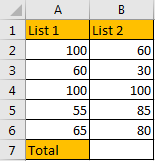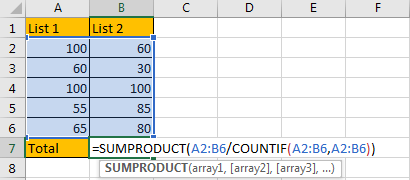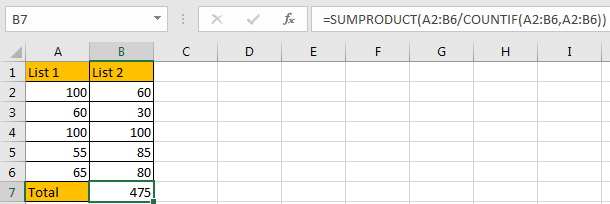# How to Sum/Calculate Total Values with Duplicate Values Only Once in Excel

In statistic when calculating the total values for a range of numbers, we often count duplicate values only once. Normally, if only apply SUM function to calculate total values, it will cover all values including all duplicate values. So, we need to update the formula to make it meet our requirement. This free tutorial will show you the formula to sum values with duplicate values only once in the selected range.

Precondition:

See screenshot below. We want to sum all values but for the duplicate values, we only count once.## Method: Calculate Total Values with Counting Duplicate Values Only Once

Step 1: In B7, enter the formula =SUMPRODUCT(A2:B6/COUNTIF(A2:B6,A2:B6)). In this formula, A2:B6 is the range selected for calculating.Step 2: Click Enter to get result. Verify that the sum value is calculated properly. Duplicate values like 100, 60 are only count once.### Related Functions

• Excel SUMPRODUCT function
The Excel SUMPRODUCT function multiplies corresponding components in the given one or more arrays or ranges, and returns the sum of those products.The syntax of the SUMPRODUCT function is as below:= SUMPRODUCT (array1,[array2],…)…
• Excel COUNTIF function
The Excel COUNTIF function will count the number of cells in a range that meet a given criteria. This function can be used to count the different kinds of cells with number, date, text values, blank, non-blanks, or containing specific characters.etc.= COUNTIF (range, criteria)…

Sidebar